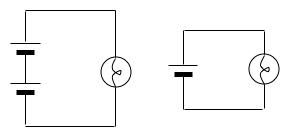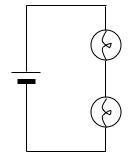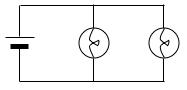Teaching Physics with the Physics Suite Edward F. Redish Problems Sorted by Type | Problems Sorted by Subject | Problems Sorted by Chapter in UP

Current flow analogies

In this problem, you will consider a number of different electric circuits and try to make sense of them using a couple of different analogies. The goal is to see if these analogies are useful for you.

The "air pressure" analogy - An electric circuit is like air flowing through a tube. The air is like movable charge, the flow of the air is like current, and the pressure in the air is like voltage.

The "loop of rope" analogy - An electric circuit is like a loop of rope going through a number of metal rings (or through pulleys or people's hands). The rope is like movable charge, its movement is like current, the rings (or pulleys or hands) are like bulbs, and tension in the rope is like voltage.

(a)(b)(c)1. If the two circuits shown at the left above (marked (a)) are set up with identical batteries and identical bulbs, the bulb on the left will be significantly brighter.   Use the two analogies to try to explain this result.
2. If the circuit shown second from the right (marked (b)) is set up with batteries and bulbs identical to the ones in A, the bulbs in this circuit will have equal brightness but be much less bright than the bulb in the circuit at the right above. Use the two analogies to try to explain this result.
3. What, if anything, would the air pressure analogy and loop of rope analogies suggest about the brightness of the bulbs at the right above (marked (c)) compared to each other and to a single-bulb circuit?

Not finding what you wanted? Check the Site Map for more information.